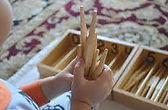top of page## ArithmeticCards and Countersthe Stamp GameCombination of Quantities & SymbolsSpindle BoxSmall Number Rods###### Philosophy:

Arithmetic is the science of computing using positive real numbers, more specifically, the process of addition, subtraction, multiplication, and division.  Little children are naturally attracted to the science of numbers.  Mathematics, like language, is the product of the human intellect and the nature of a human being.  Mathematics arises from the human mind as it comes into contact with the world and as it contemplates the universe and the factors of time and space.  It undergirds the effort of the human to understand the world in which he lives. All humans exhibit this mathematical propensity, even little children. It can therefore be said that humankind has a mathematical mind.

By age four, the child is ready for the language of mathematics.  A series of preparations have been made.  The child has established internal order, they have developed precise movement, established the work habit, and is able to follow and complete a work cycle.  The child has the ability to concentrate and has learned how to follow a process and use symbols.

The mathematical material gives the child his own mathematical experience and to arrive at individual work. There are some teacher-directed activities but these are followed by individual activities.  The Exercises in arithmetic are grouped and there is some sequential work and some parallel work.  The first group is Numbers through Ten.  The experiences in this group are sequential.  When the child has a full understanding of numbers through ten, the second group, The Decimal System, can be introduced.

The focus here is on the hierarchy of the decimal system and how the system functions. It also starts the child on the Exercises of simple computations, which are the operations of arithmetic.  The third group will be started when the decimal system is well underway.  This third group, Counting beyond Ten, includes the teens, the tens, and linear and skip counting.

The fourth group is the memorization of the arithmetic tables.  This work can begin while the later work of the decimal system and the counting beyond ten exercises are continued.

The fifth group is the passage to abstraction. The Exercises in this group require the child to understand the process of each form of arithmetic and to know the tables of each operation. There is again an overlap.  The children who know the process and tables for addition can begin to do the addition for this group.  They may still be working on learning the tables for the other operations and these will not be taken up until they have readiness.

###### Numbers to Ten
• Number Rods

• Sandpaper Numerals

• Number Rods and Number Cards

• Spindle Boxes

• Cards and Counters

• Memory Game of Numbers

Decimal System

• the Introduction to the Golden Beads

• Golden Beads - counting Through the Hierarchies

• Introduction to the Large Number Cards

• Large Number Cards - Counting Through the Hierarchies

• Identifying Large Number Cards

• Formation of Large Number Cards with Beads

• Combination of Golden Beads and Large Number Cards (Bird's Eye View)

Teens and Tens - Teens

• Formation of Quantities 11-19 with ten bars and short bead stair

• Formation of Symbols 10 - 90 with teen boards

• Combination of Quantities and Symbols to form 11-19

​Teens and Tens - Tens

• Formation of Quantities 10-99 with ten bars

• Formation of Symbols 10 - 90 with ten boards

• Combination of Quantities and Symbols 10-90 with ten bars and boards

• Formation of 11 - 99 with ten bars, unit beads, and ten boards

Teens and Tens - Counting​

• Linear Counting

• Skip Counting

Decimal System Continued​

• Changing Exercise

• Multiplication with the Golden Beads

• Subtraction with the Golden Beads

• Division with the Golden Beads

• The Stamp Game - Introductory Exercise

• The Stamp Game - Addition

• The Stamp Game - Multiplication

• The Stamp Game - Subtraction

• The Stamp Game - Division

Exploration and Memorization of Tables​

• Addition Charts 3,4,5, and 6 (blank)

• Negative Snake Game

• Negative Strip Board

• Subtraction Chart 2 and 3 (blank)

• Multiplication Board

• Multiplication chars 3, 4, and 5 (blank)

• Unit Division Board

• Division Charts 1 and 2

Passage to Abstraction​

• The Dot Game